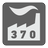Numbers Factory
Simple
English FR RU

You are given a two or more digits number N. For this mission, you should find the smallest positive number of X, such that the product of its digits is equal to N. If X does not exist, then return 0.

Let's examine the example. N = 20. We can factorize this number as 2*10, but 10 is not a digit. Also we can factorize it as 4*5 or 2*2*5. The smallest number for 2*2*5 is 225, for 4*5 -- 45. So we select 45.

... Remember prime numbers (numbers divisible by only one) and be careful with endless loops.

A number N as an integer.

The number X as an integer.

```checkio(20) == 45
checkio(21) == 37
checkio(17) == 0
checkio(33) == 0
```

This task will teach you how to work with numbers in code. You can factorize numbers and reconstruct them into new numbers. Of course you can solve this problem with brute force, but is that the best way? Numbers are the foundation of mathematics and programming.

9 < N < 10.

You should be an authorized user in order to see the full description and start solving this mission.